# KSEEB Solutions for Class 7 Maths Chapter 12 Algebraic Expressions Ex 12.1

Students can Download Class 7 Maths Chapter 12 Algebraic Expressions Ex 12.1 Questions and Answers, Notes Pdf, KSEEB Solutions for Class 7 Maths helps you to revise the complete Karnataka State Board Syllabus and to clear all their doubts, score well in final exams.

## Karnataka State Syllabus Class 7 Maths Chapter 12 Algebraic Expressions Ex 12.1

Question 1.
Get the algebraic expressions in the following cases using variables, constants and arithmetic operations
(i) Subtraction of z from y.
(ii) One-half of the sum of numbers x and y?
(iii) The number z multiplied by itself
(iv) One-fourth of the product of numbers p and q
(v) Numbers x and y both squared and added.
(vi) Number 5 added to three times the product of numbers m and n.
(vii) Product of numbersy and z subtracted from 10.
(viii) Sum of numbers a and b subtracted from their product.
Solution:
(i) y – z
(ii) $$\frac{x+y}{2}$$
(iii) z × z = z2
(iv) $$\frac{p \times q}{4}=\frac{p q}{4}$$
(v) x2 + y2
(vii) 10 – yz
(viii) ab – (a + b)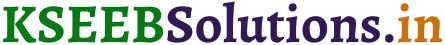Question 2.
(i) Identify the terms and their factors in the following expressions. Show the terms and factors by tree diagrams.
(a) x – 3
(b) 1 + x + x2
(c) y – y3
(d) 5xy2 + 7x2y
(e) – ab + 2b2 – 3a2
Solution:
(a) x – 3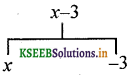(b) 1 + x + x2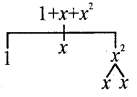(c) y – y3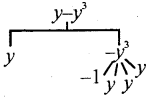(d) 5xy2 + 7x2y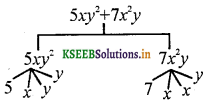(e) – ab + 2b2 – 3a2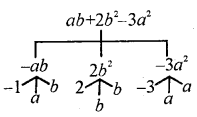(ii) Identify terms and factors in the expressions given below:
(a) – 4x + 5
(b) – 4x + 5y
(c) 5y + 3y2
(d) xy + 2x2y2
(e) pq + q
(f) 1.2 ab – 2.4 b + 3.6 a
(g) $$\frac{3}{4}$$ x + $$\frac{1}{4}$$
(h) 0.1p2 + 0.2q2
Solution: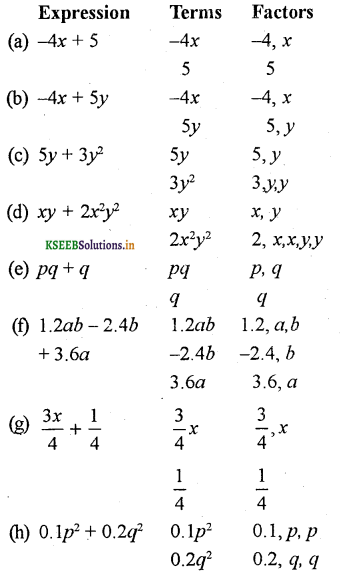Question 3.
Identify the numerical coefficients of terms (other than constants) in the following expressions:
(i) 5 – 3t2
(ii) 1 + t + t2 + t3
(iii) x + 2xy + 3y
(iv) 100m + 1000n
(v) – p2q2 + 7pq
(vi) 1.2 a + 0.8 b
(vii) 3.14 r2
(viii) 2 (l + b)
(ix) 0.1 y + 0.01y2
Solution: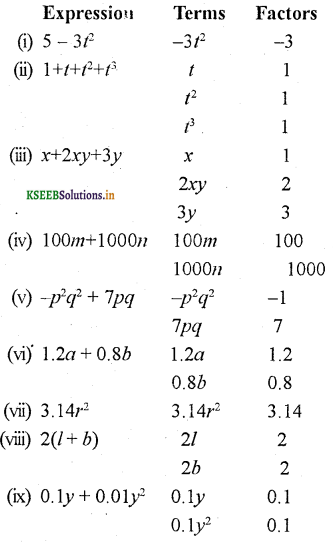Question 4.
(a) Identify terms which contain x and give the coefficient of x.
(i) y2x + y
(ii) 13y2 – 8yx
(iii) x + y + 2
(iv) 5 + z + zx
(v) 1 + x + xy
(vi) 12xy2 + 25
(vii) 7x + xy2
Solution: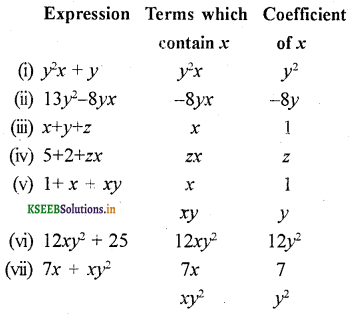(b) Identify terms which contain y2 and give the coefficient of y2.
(i) 8 – xy2
(ii) 5y2 + 7x
(iii) 2x2y – 15xy2 + 7y2
Solution: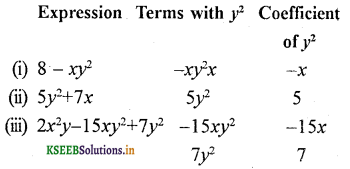Question 5.
Classify into monomials, binomials and trinomials.
(i) 4y – 7z
(ii) y2
(iii) x + y – xy
(iv) 100
(v) ab – a – b
(vi) 5 – 3t
(vii) 4p2q – 4pq2
(viii) 7mn
(ix) z2 – 3z + 8
(x) a2 + b2
(xi) z2 + z
(xii) 1 + x + x2
Solution:Question 6.
State whether a given pair of terms is of like or unlike terms.
(i) 1,100
(ii) – 7x, $$\frac{5}{2}$$x
(iii) – 29x, – 29y
(iv) 14xy, 42yx
(v) 4m2p, 4mp2
(vi) 12xz, 12x2z2
Solution:
(i) Like
(ii) Like
(iii) Unlike
(iv) Like
(v) Unlike
(vi) Unlike.

Question 7.
Identify like terms in the following:
(a) – xy2, – 4yx2, 8x2, 2xy2, 7y, – 11x2, – 100x, 11yx, 20x2y, – 6x2, y, 2xy, 3x
Solution:
– xy2, 2xy2, – 4yx2, 20x2y; 8x2, – 6x2, – 11x2, 7y, y, – 100x, 3x, – 11yx, 2xy

(b) 10pq, 7p, 8q, – p2q2, – 7qp, – 100q, – 23, 12q2p2, – 5p2, 41, 2405p, 78qp, 13p2q, qp2, 701p2
Solution:
10pq, – 7pq, 78qp, 7p, 2405p, 8q, – 100q, – p2q2, – 23, 41, – 5p2 , 701p2, 13p2q, qp2